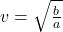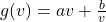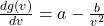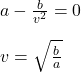## A truck uses gas as g(v)=av+bv, where v represents the speed of the truck, g represents the gallons of fuel per mile, and a and b are consta

Question

A truck uses gas as g(v)=av+bv, where v represents the speed of the truck, g represents the gallons of fuel per mile, and a and b are constants. At what speed is fuel consumption minimized?

in progress 0
5 months 2021-08-18T11:42:14+00:00 1 Answers 86 views 1Explanation:

The correct statement is:

A truck uses gas as g(v)=av+b/v, where v represents the speed of the truck and g represents the gallons of fuel per mile. At what speed is fuel consumption minimized?

You have the following function g(v):(1)

g: gallons of fuel per mile

v: speed of the truck

In order to calculate the speed of the truck, you first calculate the derivative of the g(v) respect to v:(2)

Next, you equal the previous result to zero and solve for v:For this value of v the fuel consumption is minimized.